The CANCORR Procedure
 Formulas

Assume without loss of generality that the two sets of variables,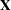with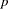variables and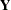withvariables, have means of zero. Letbe the number of observations, and letbe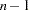.

Note that the scales of eigenvectors and canonical coefficients are arbitrary. PROC CANCORR follows the usual procedure of rescaling the canonical coefficients so that each canonical variable has a variance of one.

There are several different sets of formulas that can be used to compute the canonical correlations,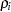,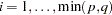, and unscaled canonical coefficients:

1. Let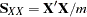be the covariance matrix of,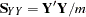be the covariance matrix of, and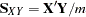be the covariance matrix betweenand. Then the eigenvalues of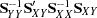are the squared canonical correlations, and the right eigenvectors are raw canonical coefficients for thevariables. The eigenvalues of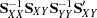are the squared canonical correlations, and the right eigenvectors are raw canonical coefficients for thevariables.

2. Let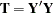and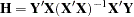. The eigenvalues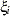of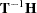are the squared canonical correlations,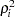, and the right eigenvectors are raw canonical coefficients for thevariables. Interchangeandin the preceding formulas, and the eigenvalues remain the same, but the right eigenvectors are raw canonical coefficients for thevariables.

3. Let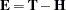. The eigenvalues of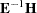are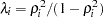. The right eigenvectors ofare the same as the right eigenvectors of.

4. Canonical correlation can be viewed as a principal component analysis of the predicted values of one set of variables from a regression on the other set of variables, in the metric of the error covariance matrix. For example, regress thevariables on thevariables. Call the predicted values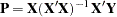and the residuals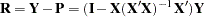. The error covariance matrix is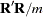. Choose a transformation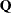that converts the error covariance matrix to an identity—that is,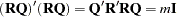. Apply the same transformation to the predicted values to yield, say,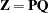. Now do a principal component analysis on the covariance matrix of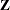, and you get the eigenvalues of. Repeat withandvariables interchanged, and you get the same eigenvalues.

To show this relationship between canonical correlation and principal components, note that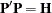,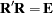, and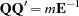. Let the covariance matrix ofbe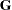. Then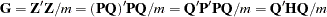. Letbe an eigenvector ofand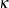be the corresponding eigenvalue. Then by definition,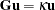; hence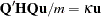. Premultiplying both sides byyields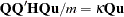and thus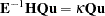. Hence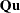is an eigenvector ofandis also an eigenvalue of.

5. If the covariance matrices are replaced by correlation matrices, the preceding formulas yield standardized canonical coefficients instead of raw canonical coefficients.

The formulas for multivariate test statistics are shown in the section Multivariate Tests in Chapter 4, Introduction to Regression Procedures. Formulas for linear regression are provided in other sections of that chapter.Previous Page | Next Page | Top of Page